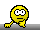## PowerTOST: sampleN.NTID() [Power / Sample Size]

Hi Helmut,

❝ Although you could give values of theta1 and theta2, for the FDA keep the defaults of 0.8 and 1.25 (i.e., don’t specify anything): Additionally to passing RSABE and the variance-comparison you must pass conventional ABE.

OK. Noted.

❝ More examples are given there.

OK. I referred these examples.

❝ See the two supportive functions in the section about dropouts in the named article.

Ok, I have pasted one example which i seems to be work upon.

❝ That’s possible if you specify a low or high theta0.

OK.

❝ Yes, you are not alone.❝ I can’t till you post an example which you consider problematic.

Note : CV is not in scalar form.'

sampleN.NTID(CV = c(0.045,0.07), theta0 = 0.95, targetpower = 0.9) +++++++++++ FDA method for NTIDs ++++++++++++            Sample size estimation --------------------------------------------- Study design:  2x2x4 (TRTR|RTRT) log-transformed data (multiplicative model) 1e+05 studies for each step simulated. alpha  = 0.05, target power = 0.9 CVw(T) = 0.045, CVw(R) = 0.07 True ratio     = 0.95 ABE limits     = 0.8 ... 1.25 Implied scABEL = 0.9290 ... 1.0764 Regulatory settings: FDA - Regulatory const. = 1.053605 - 'CVcap'           = 0.2142 Sample size search  n     power 92   0.875810 94   0.882090 96   0.888810 98   0.894060 100   0.898510 102   0.903540

# with 30% DO rate,

# as CV was specified as Vector,
# is following steps right?
balance <- function(n, n.seq) {   # Round up to obtain balanced sequences   return(as.integer(n.seq * (n %/% n.seq + as.logical(n %% n.seq)))) } nadj <- function(n, do.rate, n.seq) {   # Round up to compensate for anticipated dropout-rate   return(as.integer(balance(n / (1 - do.rate), n.seq))) } CV      <- 0.045               # Assumed CV # how to specify vector CV here? do.rate <- 0.30                # Anticipated dropout-rate 30% n       <- sampleN.NTID(CV = CV, print = FALSE, details = FALSE)[["Sample size"]] dosed   <- nadj(n, do.rate, 2) # Adjust the sample size df      <- data.frame(dosed = dosed, eligible = dosed:(n - 2)) for (j in 1:nrow(df)) {   df$dropouts[j] <- sprintf("%.1f%%", 100 * (1 - df$eligible[j] / df$dosed[j])) df$power[j]    <- suppressMessages( # We know that some are unbalanced                       power.NTID(CV = CV, n = df\$eligible[j])) } print(df, row.names = FALSE) dosed eligible dropouts   power    58       58     0.0% 0.92040    58       57     1.7% 0.91575    58       56     3.4% 0.91254    58       55     5.2% 0.90722    58       54     6.9% 0.90364    58       53     8.6% 0.89761    58       52    10.3% 0.89257    58       51    12.1% 0.88767    58       50    13.8% 0.88215    58       49    15.5% 0.87672    58       48    17.2% 0.86952    58       47    19.0% 0.86376    58       46    20.7% 0.85690    58       45    22.4% 0.84922    58       44    24.1% 0.84310    58       43    25.9% 0.83486    58       42    27.6% 0.82810    58       41    29.3% 0.81915    58       40    31.0% 0.81109    58       39    32.8% 0.80105    58       38    34.5% 0.79410

Kindly guide me with this example, i want to check whether i am making a mistake or not.Regards,
pharm07Ing. Helmut Schütz